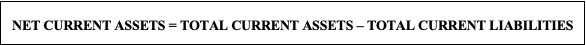## What is the formula to calculate net current assets?

Meaning of Net Current Assets In simple terms, Net Current Assets refers to the total amount of current assets excluding the total amount of current liabilities in a business. It can also be referred to as Net Working Capital. The Net Current Assets can have a positive or a negative value, wherein tRead more

# Meaning of Net Current Assets

In simple terms, Net Current Assets refers to the total amount of current assets excluding the total amount of current liabilities in a business. It can also be referred to as Net Working Capital.

The Net Current Assets can have a positive or a negative value, wherein the two are an indicator of the well-being of a business. In case the current assets are greater than the current liabilities, the company possesses sufficient assets to pay off its indebtedness and is operating efficiently. However, a company is said to be facing financial difficulty and is not in a position to pay off its debts, when the value of net current assets is negative.

The formula is as follows:## Difference between Net Current Assets and Current Assets

Net Current Assets refers to the difference between the total amount of current assets and the total amount of current liabilities whereas Current Assets are a subpart of Net Current Assets and refer to those assets that are expected to be utilized, depreciated or traded through the operations of a business within the same financial year.

For example, cash and cash equivalents, inventory, accounts receivable, etc are Current Assets whereas the summation of the same minus the total of current liabilities is termed as Net Current Assets which is further explained through an example given below.

Numerical Example

Calculate the Net Current Assets of ABC Ltd.

(Extract of Balance Sheet)

 PARTICULARS AMOUNT CURRENT ASSETS Cash and Cash Equivalents 2,00,000 Accounts Receivables 40,000 Stock Inventory 15,000 Marketable Securities 35,000 Prepaid Expenses 6,000 TOTAL CURRENT ASSETS 2,96,000 CURRENT LIABILITIES Accounts Payable 15,000 Accrued Expense 2,000 Unearned Revenue 20,000 Taxes Payable 40,000 Short-term Debt 10,000 Interest Payable 6,000 TOTAL CURRENT LIABILITIES 93,000

Solution:

Total current assets = 2,96,000

Total current liabilities= 93,000

Net Current Assets = Total Current Assets – Total Current Liabilities

= 2,96,000- 93,000  = 2,03,000

Key Takeaways

• Net Current Assets are also known as Net Working Capital.
• Net Current Assets is the difference between the total current assets and total current liabilities.
See less
• 1
• Share
Share